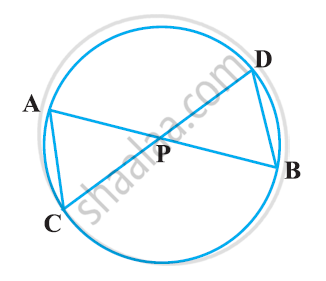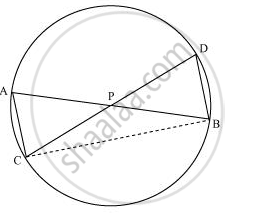Share

# In the given figure, two chords AB and CD intersect each other at the point P. prove that: (i) ΔAPC ∼ ΔDPB (ii) AP.BP = CP.DP - CBSE Class 10 - Mathematics

ConceptCriteria for Similarity of Triangles

#### Question

In the given figure, two chords AB and CD intersect each other at the point P. prove that:

(i) ΔAPC ∼ ΔDPB

(ii) AP.BP = CP.DP#### Solution

Let us join CB.(i) In ΔAPC and ΔDPB,

∠APC = ∠DPB (Vertically opposite angles)

∠CAP = ∠BDP (Angles in the same segment for chord CB)

ΔAPC ∼ ΔDPB (By AA similarity criterion)

(ii) We have already proved that

ΔAPC ∼ ΔDPB

We know that the corresponding sides of similar triangles are proportional.

:. (AP)/(DP) = (PC)/(PB) = (CA)/(BD)

=>(AP)/(DP) = (PC)/(PB)

∴ AP. PB = PC. DP

Is there an error in this question or solution?

#### APPEARS IN

NCERT Solution for Mathematics Textbook for Class 10 (2019 to Current)
Chapter 6: Triangles
Ex. 6.60 | Q: 7 | Page no. 153

#### Video TutorialsVIEW ALL 

Solution In the given figure, two chords AB and CD intersect each other at the point P. prove that: (i) ΔAPC ∼ ΔDPB (ii) AP.BP = CP.DP Concept: Criteria for Similarity of Triangles.
S